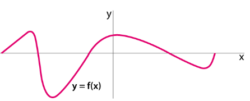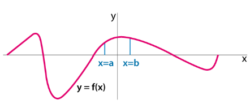# Area Under Curves Basic Concepts

Consider two people sharing an umbrella. The extent to which both of them will get drenched in the rain depends on the size of the umbrella. This can be an example of the area under the umbrella available to both of them. This article discusses the basic concepts of the area under the curves and a few solved problems.

The area under the curve can be called a change in quantity with respect to time. On integrating that, we can find the amount of quantity changed. Consider the function of x given by y = f (x). The graph below is a simple curve that can take positive, negative values depending on the value of x.The above area under the curve is unbounded in nature. If the values of x are not determined, then the answer can’t be determined.

## Important Concepts On Area Under The Curves

The area available under the curve is represented below. It is set by the limiting values of x.• The area of the curve f(x) from interval x = a to x = b, x-axis can be defined as
$$\begin{array}{l}A= \int_{a}^{b}f(x)dx\end{array}$$
. The sign is taken to be positive if the area between the two values of x lies above the x-axis and negative if the area between the two values of x lies below the x-axis.
• The area of the curve
$$\begin{array}{l}x=\phi (y)\end{array}$$
, y-axis and interval y = c to y = d is given by
$$\begin{array}{l}B=\int_{c}^{d} \phi (y)dy\end{array}$$
• If the area under one curve and another curve can be found, then the area between the curves can be calculated.

Consider two curves to be y = f (x) and y = g (x) between the interval x = a to x = b is

$$\begin{array}{l}Area=\int_{a}^{b}|f(x)-g(x)dx\end{array}$$
• The area of the ellipse =
$$\begin{array}{l}\pi*a*b\end{array}$$
• The shift in the coordinate axis leads to the area enclosed by a curve to remain invariant.
• To check the symmetry of the curve following steps are followed:
1. The curve is said to be symmetrical about the x-axis when y is changed to -y and there is no change in the curve.
2. The curve is said to be symmetrical about the y-axis when x is changed to -x and there is no change in the curve.
3. If the curve remains unchanged when x is changed to -x and y are changed to -y then it is symmetrical to both the axes.
• If x is interchanged to y results in no change of the curve, then it is symmetrical about the line y = x.

The standard steps that are followed in drawing the curve is as follows:

1] A check for symmetry is to be done.

a] Symmetry about x-axis: The curve is symmetrical about the x-axis if the curve remains unchanged when y = -y.

b] Symmetry about y-axis: The curve is symmetrical about the y-axis if the curve remains unchanged when x = -x.

c] Symmetrical about both axes: The curve is symmetrical about both axes if the curve remains unchanged when x = -x and y = -y.

d] Symmetrical about the line y = x: The curve is said to be symmetrical about the line y = x if the curve remains unchanged on interchanging x and y.

2] The point of intersection of the axes is to be noted.

3] Find dy / dx to find stationary points. Find the intervals to determine whether the function is increasing or decreasing.

4] Find y when x → ∞ or -∞.

Integration

Definite and indefinite integration

Indefinite Integrals Problems and Solutions

## How to Find the Area Under Curves

Few examples are illustrated below on finding areas enclosed by different curves.

Example 1: What is the area bounded by the curve y = logx, x−axis and the ordinates x = 1, x = 2?

Solution:

Given curve y = logx and x = 1, x = 2

Hence required area =[

$$\begin{array}{l}\int_{1}^{2}logxdx=(x\ logx-x)\end{array}$$
]21

= 2 log2 − 1 = (log 4 − 1) sq. unit

Example 2: If the ordinate x = a divides the area bounded by the curve y = (1 + [8 / x2]), the x−axis and the ordinates x = 2, x = 4 into two equal parts, then what is the value of a?

Solution:

Let the ordinate at x = a divide the area into two equal parts.

$$\begin{array}{l}AMNB=\int_{4}^{2}=[1+\frac{8}{x^2}]dx=[x-\frac{8}{x}]{_{2}}^{4}=4\end{array}$$
and

$$\begin{array}{l}ACDM=\int_{2}^{a}=[1+\frac{8}{x^2}]dx=2\end{array}$$

On solving, we get a=± 2√2

Since a>0, a=2√2

Example 3: If area bounded by the curves y2 = 4ax and y = mx is a2 / 3, then find the value of m.

Solution:

The two curves y2 = 4ax and y = mx intersect at (4a / m2, 4a / m) and the area enclosed by the two curves is given by

$$\begin{array}{l}\int_{0}^{4a/m^2}[\sqrt{4ax-mx}]dx=\frac{a^2}{3}\end{array}$$
[8 / 3] * [a2 / m3] = a2/ 3

⇒ m3 = 8

⇒ m = 2

Example 4: Find the ratio of the areas bounded by the curves y = cosx and y = cos2x between x = 0, x = π/3 and x−axis.

Solution:

$$\begin{array}{l}A_1=\int_{0}^{\pi/3}cos\ x\ dx=\frac{\sqrt{3}}{2}\end{array}$$
$$\begin{array}{l}A_2=\int_{0}^{\pi/3}cos2x dx=\frac{\sqrt{3}}{4}\end{array}$$

Hence the ratio is A1 : A2 = 2 : 1

Example 5: What is the area of smaller part between the circle x2 + y2 = 4 and the line x = 1.

Solution:

Area of smaller part =

$$\begin{array}{l}2\int_{1}^{2}\sqrt{4-x^2}dx=2[\frac{x}{2}\sqrt{4-x^2}+2sin^{-1}\frac{x}{2}]\end{array}$$
in the interval [1,2]

=

$$\begin{array}{l}2[2*\frac{\pi}{2}-[\frac{\sqrt{3}}{2}-2*\frac{\pi}{6}]\end{array}$$

=

$$\begin{array}{l}2[\pi-[\frac{\sqrt{3}}{2}-\frac{\pi}{3}]\end{array}$$

=

$$\begin{array}{l}\frac{8\pi}{3}-\sqrt{3}\end{array}$$

Example 6: What isthe area in the first quadrant between x2 + y2 = π2 and y = sinx?

Solution:

The area of the circle in the first quadrant is π* (π2) / 4 i.e., π3 / 4.

Also, the area bounded by curve y = sinx and x-axis is 2 sq. unit.

Hence, required area is [π3 / 4] − 2 = [π3 − 8] / 4

Example 7: The area enclosed by the curves y = sinx + cosx and y = |cosx – sinx| over the interval

$$\begin{array}{l}[0,\frac{\pi}{2}]\end{array}$$
is

Solution:

$$\begin{array}{l}y_{1}=sinx+cosx=\sqrt{2}sin(x+\frac{\pi}{4})\\ y_{2}=|sinx-cosx|=\sqrt{2}sin|sin\frac{\pi}{4}-x|\\ \text \ Area =\int_{0}^\frac{\pi}{4}[(sinx+cosx)-(cosx-sinx)]dx+\int_{\frac{\pi}{4}}^{\frac{\pi}{2}}[(sinx+cosx)-(sinx-cosx)]dx\\ =4-2\sqrt{2}\\ =2\sqrt{2}(\sqrt{2}-1)\end{array}$$

Example 8: Let

$$\begin{array}{l}g(x)=cosx^{2},f(x)=\sqrt{x} \text \ and \ \alpha , \beta (\alpha <\beta )\end{array}$$
be the roots of the quadratic equation
$$\begin{array}{l}18x^2-9\pi x+{\pi}^{2}=0\end{array}$$
. Then the area bounded by the curve y = (gof) (x) and the lines
$$\begin{array}{l}x=\alpha , x=\beta \text \ and \ y= 0\end{array}$$
is

Solution:

$$\begin{array}{l}18x^2-9\pi x+{\pi}^{2}=0 ; gof(x)=cosx\\(3x-\pi)(6x-\pi)=0\\\alpha =\frac{\pi}{6}, \beta =\frac{\pi}{3}\\A=\int_{\frac{\pi}{6}}^{\frac{\pi}{3}}cosxdx=\frac{\sqrt{3}-1}{2}\end{array}$$

Example 9: Say

$$\begin{array}{l}A_{n}\end{array}$$
be the area bounded by the curve
$$\begin{array}{l}=({tanx})^{n}\end{array}$$
and the lines
$$\begin{array}{l}x=0,y=0,x=\frac{\pi}{4}\end{array}$$
. Show that

$$\begin{array}{l}a] \ A_{n}+A_{n-2}=\frac{1}{n-1}\\ b] \ \frac{1}{2n+2}<A_{n}<\frac{1}{2n-2} \forall n\end{array}$$

Solution:

Area bounded by the

$$\begin{array}{l}=({tanx})^{n}\end{array}$$
and the lines
$$\begin{array}{l}x=0,y=0,x=\frac{\pi}{4}\end{array}$$
is

$$\begin{array}{l}A_{n}=\int_{0}^{\frac{\pi}{4}}(tan^{n}x)dx\\ A_{n}=\int_{0}^{\frac{\pi}{4}}(tan^{n-2}x) *(sec^{2}x-1)dx\\ A_{n}=\frac{1}{n-1}-0-A_{n-2}\\ A_{n}+A_{n-2}=\frac{1}{n-1}\rightarrow (1)\\\text \ In \ (0,\frac{\pi}{4}), \int_{0}^{\frac{\pi}{4}} (tan^{n}x)dx<\int_{0}^{\frac{\pi}{4}}(tan^{n-2}x)dx\\\text \ So \ A_{n}<A_{n-2} \text \ and \ A_{n+2}<A_{n}\\A_{n}+A_{n+2}<2A_{n}\\2A_{n} < \frac{1}{n-2} \text \ and \ \frac{1}{(n+1)} < 2 A_{n}\\\frac{1}{(n+1)} < 2A_{n} < \frac{1}{n-2}\\\frac{1}{2n+2}<A_{n}<\frac{1}{2n-2} \forall n\end{array}$$

## Applications of Integrals Videos

### Definite Integral & Area Under the Curve – Important Topics### Definite Integral & Area Under the Curve – Important Questions## Frequently Asked Questions

### Give the formula to find the area under the curve with respect to x-axis.

The area under the curve y = f(x) and x-axis is given by the equation A = ∫ab f(x) dx. Here a and b are the bounding values for the curve with respect to the x-axis.

### Give the formula to find the area under the curve with respect to the y-axis.

The area of the curve bounded by the curve x = f(y), the y-axis, across the lines y = a and y = b is given by the equation A = ∫ab f(y) dy.

### What is the area of an ellipse?

Area of an ellipse is given by A = πab.

### How to find the area under a curve?

Using the integration or antiderivative, we can find the area under the curve. We need the equation of the curve y = f(x), the axis bounding the curve, and the boundary limits of the curve.